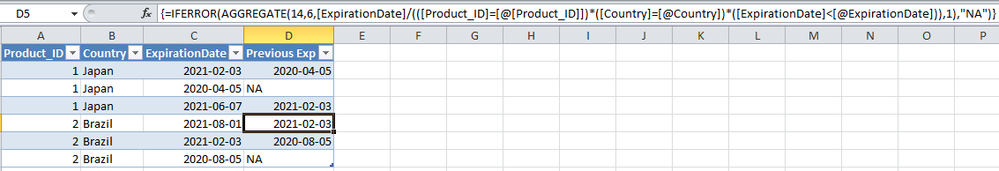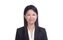cancel
Showing results for
Did you mean:Frequent Visitor

## Get the previous date from same table to add into visual

Hello there,

I am trying to populate a column in a table to get the previous expiration date (most recent) within the same table.In the first row, I need to filter the same table where the Product ID and Country Match with this first row and populate the previous expiration date. There may be more than two or three of the same Product ID and Country, with different expiration date, but I only need to obtain the most recent one only.

In the second row, the column will return Not applicable since there is no previous expiration date that match with the Product ID and Country.

In the third row, has the same logic as the first row.

I will be using this value into a table visual so we can export into excel.

I tried to use the solution here below, but I just got the latest date in the table 8/1/2021:

https://community.powerbi.com/t5/Desktop/Values-from-previous-transaction-date/m-p/1548914#M633624

been trying out different method for a couple days, but cannot yet figure it out. Please help!

2 ACCEPTED SOLUTIONSSuper User

@HenWib , as to me, calculated column or PQ would be easy to do the trick,

PQ solution,

``````let
Source = Table.FromRows(Json.Document(Binary.Decompress(Binary.FromText("i45WMlTSUfJKLEjMA9JGBkaGugZGugbGSrE6GFIGugYmugam2KSAusx0DczBUkZAIaeixKrMHLicha6BIS45uG3ocgZgfUDrYgE=", BinaryEncoding.Base64), Compression.Deflate)), let _t = ((type nullable text) meta [Serialized.Text = true]) in type table [Product_ID = _t, Country = _t, ExpirationDate = _t]),
#"Changed Type" = Table.TransformColumnTypes(Source,{{"Product_ID", Int64.Type}, {"Country", type text}, {"ExpirationDate", type date}}),

#"Changed Type",
"Previous Exp PQ",
each
let
dates = Table.Group(#"Changed Type", {"Product_ID", "Country"}, {"Grouped", each _}){[Product_ID=[Product_ID], Country=[Country]]}[Grouped][ExpirationDate],
res = List.Max(List.Select(dates, (x)=> x<[ExpirationDate]))??"NA"
in res
)
in

Calculated column solution,

``````Previous Exp CC =
MAXX (
FILTER (
'Table1',
'Table1'[Product_ID] = EARLIER ( 'Table1'[Product_ID] )
&& 'Table1'[Country] = EARLIER ( 'Table1'[Country] )
&& 'Table1'[ExpirationDate] < EARLIER ( 'Table1'[ExpirationDate] )
),
'Table1'[ExpirationDate]
)``````

Of coz, DAX is capable of solving it with ease; but it's subject to columns in the viz,

``````Previous Exp M =
VAR __dd = MAX ( 'Table1'[ExpirationDate] )
RETURN
MAXX (
FILTER (
CALCULATETABLE (
'Table1',
ALLEXCEPT ( 'Table1', 'Table1'[Product_ID], 'Table1'[Country] )
),
'Table1'[ExpirationDate] < __dd
),
'Table1'[ExpirationDate]
)``````For fun, Excel array formula, our oldie but goodie, also does the trick.

``{=IFERROR(AGGREGATE(14,6,[ExpirationDate]/(([Product_ID]=[@[Product_ID]])*([Country]=[@Country])*([ExpirationDate]<[@ExpirationDate])),1),"NA")}``Thanks to the great efforts by MS engineers to simplify syntax of DAX! Most beginners are SUCCESSFULLY MISLED to think that they could easily master DAX; but it turns out that the intricacy of the most frequently used RANKX() is still way beyond their comprehension! DAX is simple, but NOT EASY!Community Support

Hi, @HenWib

Based on your description, I created data to reproduce your scenario. The pbix file is attached in the end.

Table:You may create a calculated column or a measure as below.

Calculated column:

``````Previous Expiration Date Column =
CALCULATE(
MAX('Table'[ExpirationDate]),
FILTER(
ALL('Table'),
[Product_ID]=EARLIER('Table'[Product_ID])&&
[Country]=EARLIER('Table'[Country])&&
[ExpirationDate]<EARLIER('Table'[ExpirationDate])
)
)``````

Measure:

``````Previous Expiration Date Measure =
CALCULATE(
MAX('Table'[ExpirationDate]),
FILTER(
ALL('Table'),
[Product_ID]=MAX('Table'[Product_ID])&&
[Country]=MAX('Table'[Country])&&
[ExpirationDate]<MAX('Table'[ExpirationDate])
)
)``````

Result:Best Regards

Allan

If this post helps, then please consider Accept it as the solution to help the other members find it more quickly.

3 REPLIES 3Community Support

Hi, @HenWib

Based on your description, I created data to reproduce your scenario. The pbix file is attached in the end.

Table:You may create a calculated column or a measure as below.

Calculated column:

``````Previous Expiration Date Column =
CALCULATE(
MAX('Table'[ExpirationDate]),
FILTER(
ALL('Table'),
[Product_ID]=EARLIER('Table'[Product_ID])&&
[Country]=EARLIER('Table'[Country])&&
[ExpirationDate]<EARLIER('Table'[ExpirationDate])
)
)``````

Measure:

``````Previous Expiration Date Measure =
CALCULATE(
MAX('Table'[ExpirationDate]),
FILTER(
ALL('Table'),
[Product_ID]=MAX('Table'[Product_ID])&&
[Country]=MAX('Table'[Country])&&
[ExpirationDate]<MAX('Table'[ExpirationDate])
)
)``````

Result:Best Regards

Allan

If this post helps, then please consider Accept it as the solution to help the other members find it more quickly.Super User

@HenWib , as to me, calculated column or PQ would be easy to do the trick,

PQ solution,

``````let
Source = Table.FromRows(Json.Document(Binary.Decompress(Binary.FromText("i45WMlTSUfJKLEjMA9JGBkaGugZGugbGSrE6GFIGugYmugam2KSAusx0DczBUkZAIaeixKrMHLicha6BIS45uG3ocgZgfUDrYgE=", BinaryEncoding.Base64), Compression.Deflate)), let _t = ((type nullable text) meta [Serialized.Text = true]) in type table [Product_ID = _t, Country = _t, ExpirationDate = _t]),
#"Changed Type" = Table.TransformColumnTypes(Source,{{"Product_ID", Int64.Type}, {"Country", type text}, {"ExpirationDate", type date}}),

#"Changed Type",
"Previous Exp PQ",
each
let
dates = Table.Group(#"Changed Type", {"Product_ID", "Country"}, {"Grouped", each _}){[Product_ID=[Product_ID], Country=[Country]]}[Grouped][ExpirationDate],
res = List.Max(List.Select(dates, (x)=> x<[ExpirationDate]))??"NA"
in res
)
in

Calculated column solution,

``````Previous Exp CC =
MAXX (
FILTER (
'Table1',
'Table1'[Product_ID] = EARLIER ( 'Table1'[Product_ID] )
&& 'Table1'[Country] = EARLIER ( 'Table1'[Country] )
&& 'Table1'[ExpirationDate] < EARLIER ( 'Table1'[ExpirationDate] )
),
'Table1'[ExpirationDate]
)``````

Of coz, DAX is capable of solving it with ease; but it's subject to columns in the viz,

``````Previous Exp M =
VAR __dd = MAX ( 'Table1'[ExpirationDate] )
RETURN
MAXX (
FILTER (
CALCULATETABLE (
'Table1',
ALLEXCEPT ( 'Table1', 'Table1'[Product_ID], 'Table1'[Country] )
),
'Table1'[ExpirationDate] < __dd
),
'Table1'[ExpirationDate]
)``````For fun, Excel array formula, our oldie but goodie, also does the trick.

``{=IFERROR(AGGREGATE(14,6,[ExpirationDate]/(([Product_ID]=[@[Product_ID]])*([Country]=[@Country])*([ExpirationDate]<[@ExpirationDate])),1),"NA")}``Thanks to the great efforts by MS engineers to simplify syntax of DAX! Most beginners are SUCCESSFULLY MISLED to think that they could easily master DAX; but it turns out that the intricacy of the most frequently used RANKX() is still way beyond their comprehension! DAX is simple, but NOT EASY!Super User

Hi @HenWib

Are you looking for a measure, or a calculated column in DAX or a column in M? Here is a measure:

Previous Date =
VAR curDate = SELECTEDVALUE('Table'[ExpirationDate])
VAR T1 = FILTER(ALL('Table'),[ExpirationDate]<curDate)
RETURN
COALESCE(MAXX(T1,[ExpirationDate]),"NA")